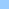# CodeCounter

count the number of lines of lingo code
LingolinesCodeCounter ``` ----------------------------------------------------------- -- nach einer Anregung von "Arturo Batanero" on CodeCounter lib --Calcs set LineCount = [0,0,0] set ini = iif(voidP(lib), 1, lib) set end = iif(voidP(lib), the number of castlibs, ini) repeat with i = ini to end set lastMbr = the number of members of castLib i repeat with j = 1 to lastMbr if the scripttext of member j of castlib i > "" then set LineCount = LineCount + ¬ CountCodeLines(the scriptText of member j of castlib i) end if end repeat end repeat --Reports -- avoid a script error if voidP(lib) set lib = iif(voidP(lib),1,lib) if ini=end then put "Lingo lines in castlib '" & ¬ the name of castlib lib & "'(#" & lib & ")" else put "Lingo lines in all castlibs" set CodeOnly = GetAt(LineCount, 1) set Comments = GetAt(LineCount, 2) set Total = GetAt(LineCount, 3) set Blanks = Total - (CodeOnly + Comments) put " Only code:" && CodeOnly put " Only comments:" && Comments put " Code&comments:" && (CodeOnly + Comments) put " Blank lines:" && Blanks put " Total lines:" && Total put "Comment % of Code:" && Comments * 100 / CodeOnly put "Blanks % of Code:" && (Total - (CodeOnly + Comments)) * 100 / CodeOnly end on CountCodeLines script set CodeOnly = 0 set CommentOnly = 0 set TotalLines = the number of lines of script -- repeat with i = 1 to TotalLines set currLine = word 1 of line i of script if currLine <> EMPTY then if (chars(currLine,1,2)<>"--") then set CodeOnly = CodeOnly + 1 else set CommentOnly = CommentOnly + 1 end if end repeat return list(CodeOnly, CommentOnly, TotalLines) end --This is a general purpose function for "the-shortest- --the-better" lovers. It allows very compact inline --conditional sentences. --If you know VisualBasic then i'm sure you will feel --at home. -- --Example: instead of this kind of code: -- if n>2 then -- set size = "It's Bigger" -- else -- set size = "It's Smaller" -- end if -- --Now you just have to write this: -- set sz = "It's " & iif(n>2, "Bigger", "Smaller") -- on iif evalExpr, ExprTrue, ExprFalse if evalExpr then return ExprTrue else Return ExprFalse end ```Home shock + cgi Bits 'n pieces Director Lingo ShockLets Contact